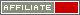# Fast loss recovery in cycles

This trigger was suggested on the forum.

I've summarised it below.

Lay on "2 Card run" in HiLo in the first round. If the bet has lost, calculate the next bet according to the formula:

([loss to recoup] + [initial stake] * ([number of losses in a row] + [cycles]))/[cycles]

Here [loss to recoup] is the cumulative loss from the previous bets that needs to be recouped. Then [initial stake] is the amount of the very first bet, [number of losses in a row] is the number of consecutive times the game has ended in loss (without any winning games in between). Finally, [cycles] is the number of steps in which the loss will be recouped. The less is this number, the bigger will be the stakes.

Example: initial stake is £4, cycles = 2.

I lay £4 at 2.07 and lose £4.28. I have lost one time so far, so the next bet will be calculated as (4.28 + 4 * (1 + 2))/2 = £8.14.

So I lay £8.14 two times in a row, and provided both bets have won, I will have my loss recouped, and will return to the initial bet size of £4.

If, however, the first £8.14 bet has lost too (-8.71), the cumulative loss to recoup becomes 4.28 + 8.71 = 12.99, so my next bet will be:

(12.99 + 4*(2 + 2))/2 = 14.5

and so on, until the winning sequence of two bets.

If the first bet of 8.14 has won, yet the second one has lost, I deduct the amount won by the first bet from the loss that needs to be recouped and repeat the same procedure.

The aim of this plan is to win exactly one unit per each game.© 2005-2020 WellDone Creative Software. All rights reserved. Terms and conditions.